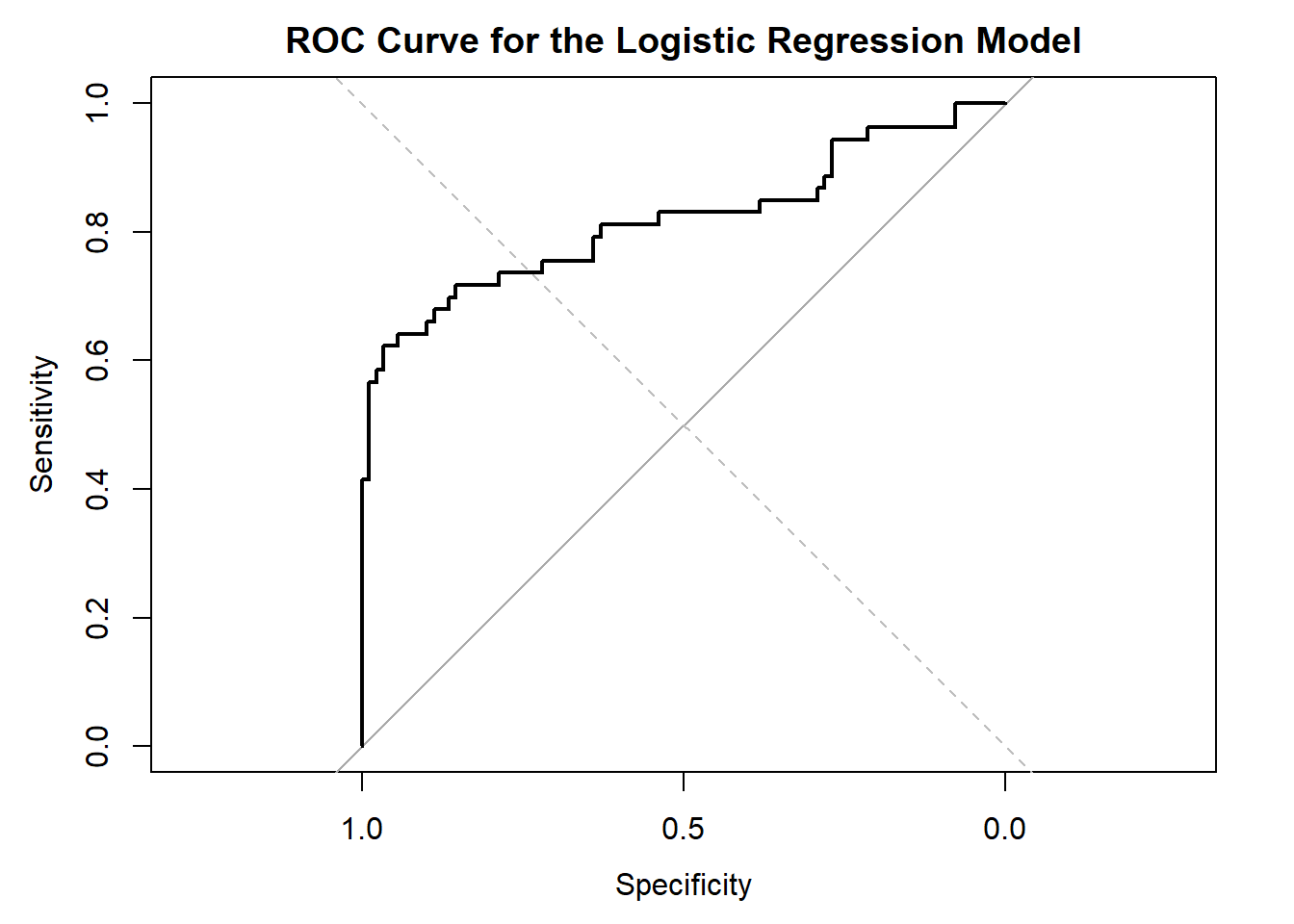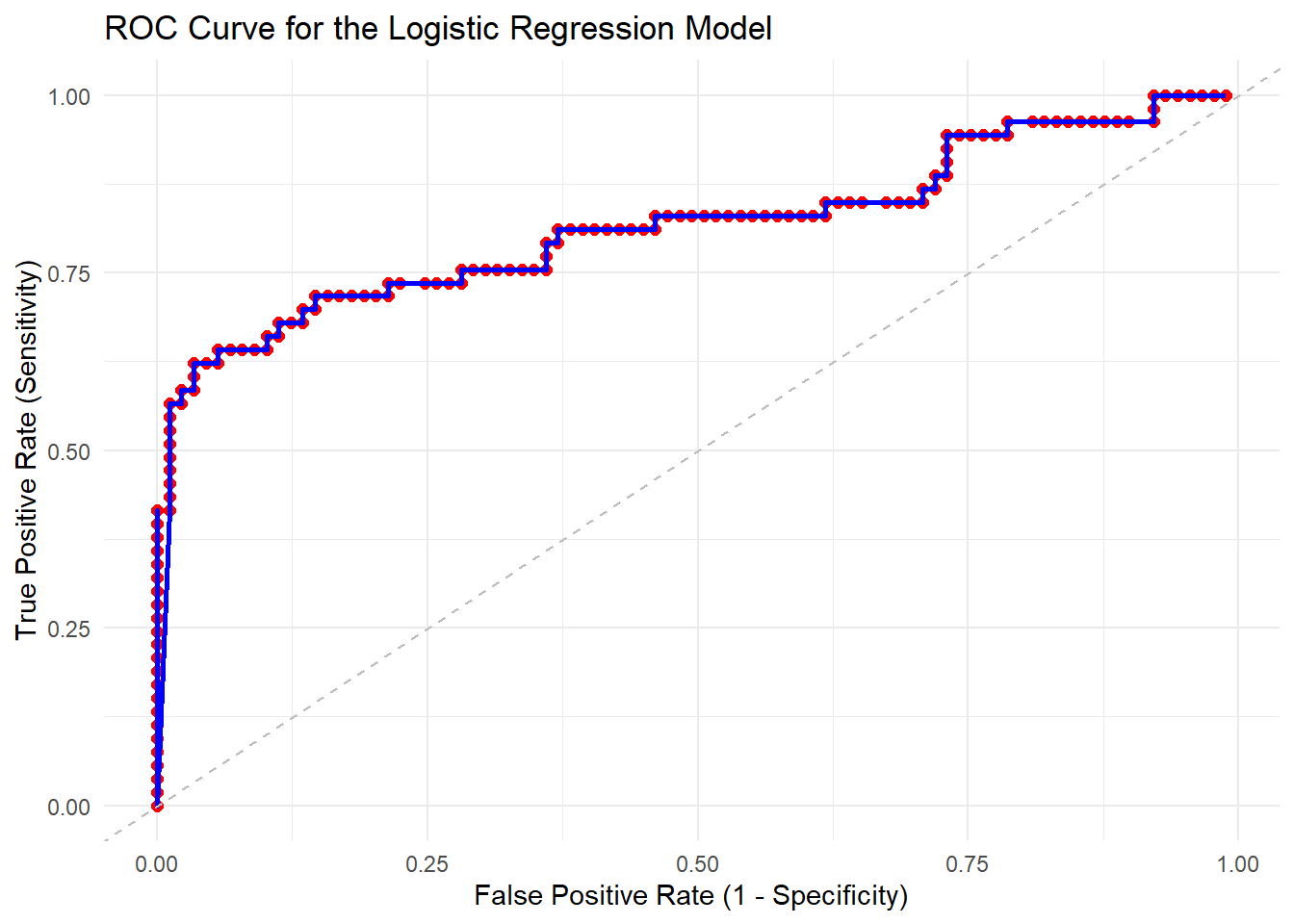# Understanding the Confusion Matrix and ROC Curve in R

혼동이 찾아오는 혼돈행렬

R
ML
Author

Changjun Lee

Published

March 21, 2023

## Introduction

In this blog post, we will explore two essential concepts in evaluating classification models: the confusion matrix and the Receiver Operating Characteristic (ROC) curve. We will go through the basics, discuss how to interpret these metrics, and provide R code snippets to create and visualize them using the popular `caret` and `pROC` packages. We’ll demonstrate these concepts using the Titanic dataset.

The Titanic dataset is not available in R by default, but it can be loaded using the `titanic` package. First, install and load the package:

``````# Install the titanic package if you haven't already
# if (!requireNamespace("titanic", quietly = TRUE)) {
#   install.packages("titanic")
# }

library(titanic)
library(tidyverse)``````
``````── Attaching core tidyverse packages ──────────────────────── tidyverse 2.0.0 ──
✔ dplyr     1.1.3     ✔ readr     2.1.4
✔ forcats   1.0.0     ✔ stringr   1.5.0
✔ ggplot2   3.4.4     ✔ tibble    3.2.1
✔ lubridate 1.9.3     ✔ tidyr     1.3.0
✔ purrr     1.0.2
── Conflicts ────────────────────────────────────────── tidyverse_conflicts() ──
ℹ Use the conflicted package (<http://conflicted.r-lib.org/>) to force all conflicts to become errors``````
``````# Load the Titanic dataset
data("titanic_train")
data("titanic_test")

# Combine the training and testing datasets
titanic_data <- bind_rows(titanic_train, titanic_test)``````

Before we proceed, let’s preprocess the dataset by selecting relevant features and handling missing values:

``````# Select relevant features and remove rows with missing values
titanic_data <- titanic_data[, c("Survived", "Pclass", "Sex", "Age", "SibSp", "Parch", "Fare")]
titanic_data <- na.omit(titanic_data)

# Convert the 'Sex' variable to a factor
titanic_data\$Sex <- as.factor(titanic_data\$Sex)``````

## Confusion Matrix

A confusion matrix is a tabular representation of the predictions made by a classification model, showing the number of correct and incorrect predictions for each class. It is a useful tool to evaluate a model’s performance and identify its strengths and weaknesses.

### Creating a Confusion Matrix in R

To demonstrate the confusion matrix, we will use the preprocessed Titanic dataset and create a logistic regression model. First, let’s load the required packages and split the dataset into training (80%) and testing (20%) sets:

``````# Load required packages
library(caret)``````
``Loading required package: lattice``
``````
Attaching package: 'caret'``````
``````The following object is masked from 'package:purrr':

lift``````
``````# Split the dataset into training (80%) and testing (20%) sets
set.seed(42)
train_index <- createDataPartition(titanic_data\$Survived, p = 0.8, list = FALSE)
titanic_train <- titanic_data[train_index, ]
titanic_test <- titanic_data[-train_index, ]``````

Now, let’s create a logistic regression model and make predictions on the test set:

``````# Create the logistic regression model
model <- glm(Survived ~ ., data = titanic_train, family = "binomial")

# Make predictions on the test dataset
predicted_probs <- predict(model, titanic_test, type = "response")
predicted_classes <- ifelse(predicted_probs > 0.5, 1, 0)``````

Next, we will create the confusion matrix using the `caret` package:

``````# Create the confusion matrix
cm <- confusionMatrix(table(predicted_classes, titanic_test\$Survived))
print(cm)``````
``````Confusion Matrix and Statistics

predicted_classes  0  1
0 78 17
1 11 36

Accuracy : 0.8028
95% CI : (0.7278, 0.8648)
No Information Rate : 0.6268
P-Value [Acc > NIR] : 4.43e-06

Kappa : 0.5687

Mcnemar's Test P-Value : 0.3447

Sensitivity : 0.8764
Specificity : 0.6792
Pos Pred Value : 0.8211
Neg Pred Value : 0.7660
Prevalence : 0.6268
Detection Rate : 0.5493
Detection Prevalence : 0.6690
Balanced Accuracy : 0.7778

'Positive' Class : 0
``````

### Interpreting the Confusion Matrix

The confusion matrix displays the following information:

• `True Positives (TP)`: 78, the number of correctly predicted non-survivors (class 0)

• `True Negatives (TN)`: 36, the number of correctly predicted survivors (class 1)

• `False Negatives (FN)`: 11, the number of non-survivors incorrectly predicted as survivors (class 1)

• `False Positives (FP)`: 17, the number of survivors incorrectly predicted as non-survivors (class 0)

Statistics:

• `Accuracy`: 0.8028 (80.28%), the proportion of correct predictions (both true positives and true negatives) among the total number of cases. The 95% CI (confidence interval) for the accuracy is (0.7278, 0.8648), meaning we can be 95% confident that the true accuracy lies within this range.

• `No Information Rate (NIR)`: 0.6268, the accuracy that could be obtained by always predicting the majority class (class 0 in this case).

• `P-Value [Acc > NIR]`: 4.43e-06, the p-value for a statistical test comparing the accuracy of the model to the NIR. A small p-value (typically less than 0.05) indicates that the model’s accuracy is significantly better than the NIR.

• `Kappa`: 0.5687, a metric that considers both the true positive rate and the false positive rate, providing a more balanced assessment of the model’s performance. Kappa ranges from -1 to 1, with 0 indicating no better than random chance, and 1 indicating perfect agreement between predictions and true values.

• `Mcnemar's Test P-Value`: 0.3447, the p-value for a statistical test comparing the number of false positives and false negatives. A large p-value (typically greater than 0.05) indicates that there is no significant difference between the number of false positives and false negatives.

Sensitivity, Specificity, and Other Metrics:

• `Sensitivity` (Recall or True Positive Rate): 0.8764, the proportion of actual positive cases (survivors) that were correctly identified by the model.

• `Specificity`: 0.6792, the proportion of actual negative cases (non-survivors) that were correctly identified by the model.

• `Positive Predictive Value (PPV)`: 0.8211, the proportion of positive predictions (predicted survivors) that were actually positive (true survivors).

• `Negative Predictive Value (NPV)`: 0.7660, the proportion of negative predictions (predicted non-survivors) that were actually negative (true non-survivors).

• `Prevalence`: 0.6268, the proportion of the true positive cases (survivors) in the dataset.

• `Detection Rate`: 0.5493, the proportion of true positive cases that were correctly detected by the model.

• `Detection Prevalence`: 0.6690, the proportion of cases predicted as positive (survivors) by the model.

• `Balanced Accuracy`: 0.7778, the average of sensitivity and specificity, providing a balanced assessment of the model’s performance across both classes.

The ‘Positive’ class is set to 0 (non-survivors) in this analysis.

## ROC Curve

The Receiver Operating Characteristic (ROC) curve is a graphical representation of a classifier’s performance across all possible decision thresholds. It plots the True Positive Rate (TPR, also known as sensitivity or recall) against the False Positive Rate (FPR, or 1 - specificity) at various threshold settings.

### Creating an ROC Curve in R

To create an ROC curve, we will use the `pROC` package. First, let’s install and load the required package:

``````# Install the pROC package if you haven't already
# if (!requireNamespace("pROC", quietly = TRUE)) {
#   install.packages("pROC")
# }

library(pROC)``````
``Type 'citation("pROC")' for a citation.``
``````
Attaching package: 'pROC'``````
``````The following objects are masked from 'package:stats':

cov, smooth, var``````

Now, let’s create the ROC curve using the predicted probabilities from our logistic regression model:

``````# Create the ROC curve
roc_obj <- roc(titanic_test\$Survived, predicted_probs)``````
``Setting levels: control = 0, case = 1``
``Setting direction: controls < cases``
``````# Plot the ROC curve
plot(roc_obj, main = "ROC Curve for the Logistic Regression Model")
abline(0, 1, lty = 2, col = "gray")  # Add a reference line for a random classifier``````### Interpreting the ROC Curve

The ROC curve helps us visualize the trade-off between sensitivity (True Positive Rate) and specificity (1 - False Positive Rate) for various threshold values. A perfect classifier would have an ROC curve that passes through the top-left corner of the plot (100% sensitivity and 100% specificity). In contrast, a random classifier would have an ROC curve that follows the diagonal reference line (gray dashed line in our plot).

The area under the ROC curve (AUC) is a scalar value that summarizes the performance of the classifier. An AUC of 1.0 indicates a perfect classifier, while an AUC of 0.5 suggests that the classifier is no better than random chance. We can calculate the AUC using the `auc` function from the `pROC` package:

``````# Calculate the AUC
auc_value <- auc(roc_obj)
cat("AUC:", auc_value, "\n")``````
``AUC: 0.8181047 ``

Let’s go through the process of drawing dots and lines in the ROC curve step by step.

1. Understand the components of the ROC curve: The ROC curve consists of several points (dots) that represent the true positive rate (sensitivity) and false positive rate (1 - specificity) at various decision thresholds. To draw the curve, you need to connect these points with lines.

2. Determine decision thresholds: You must first identify the decision thresholds you want to use. These thresholds represent the probability cut-off points for classifying an observation as positive or negative. In most cases, you can use the unique predicted probabilities in your dataset as the thresholds.

``````# Extract unique predicted probabilities
thresholds <- unique(predicted_probs)

# Sort the thresholds in descending order
thresholds <- sort(thresholds, decreasing = TRUE)``````
1. Calculate TPR and FPR for each threshold: For each threshold, calculate the true positive rate (sensitivity) and false positive rate (1 - specificity).
``````# Initialize empty vectors for TPR and FPR
tpr <- numeric(length(thresholds))
fpr <- numeric(length(thresholds))

# Calculate TPR and FPR for each threshold
for (i in seq_along(thresholds)) {

threshold <- thresholds[i]

# Classify observations based on the current threshold
predicted_classes <- as.integer(predicted_probs >= threshold)

# Create a confusion matrix
cm <- table(Predicted = predicted_classes,
Actual = titanic_test\$Survived)

# Calculate TPR and FPR
if(sum(dim(cm))==4){
tpr[i] <- cm["1", "1"] / (cm["1", "1"] + cm["0", "1"])
fpr[i] <- cm["1", "0"] / (cm["1", "0"] + cm["0", "0"])
}

}``````
1. Plot the ROC curve: Now that you have calculated the TPR and FPR for each threshold, you can plot the ROC curve by connecting the dots (points) with lines.
``````# Create a data frame for plotting
roc_df <- data.frame(Threshold = thresholds, TPR = tpr, FPR = fpr)

# Create the ROC plot
roc_plot <- ggplot(roc_df, aes(x = FPR, y = TPR)) +
geom_point(size = 2, color = "red") +  # Add points (dots)
geom_line(color = "blue", size = 1) +  # Connect the points with lines
geom_abline(intercept = 0, slope = 1, linetype = "dashed", color = "gray") +
labs(
title = "ROC Curve for the Logistic Regression Model",
x = "False Positive Rate (1 - Specificity)",
y = "True Positive Rate (Sensitivity)"
) +
theme_minimal()``````
``````Warning: Using `size` aesthetic for lines was deprecated in ggplot2 3.4.0.
``````# Display the plot
print(roc_plot)``````This will create an ROC curve with dots (points) representing each decision threshold and lines connecting these dots. The resulting plot allows you to visualize the trade-offs between sensitivity and specificity at various threshold settings.

Let’s see the process of drawing the ROC curve step by step.

``````library(gganimate)

animated_roc_plot <- ggplot(roc_df, aes(x = FPR, y = TPR)) +
geom_point(aes(group = seq_along(Threshold),
color = as.factor(seq_along(Threshold))),
size = 2, show.legend = FALSE) +
geom_line(aes(group = seq_along(Threshold),
color = as.factor(seq_along(Threshold))),
size = 1, show.legend = FALSE) +
geom_abline(intercept = 0, slope = 1, linetype = "dashed", color = "gray") +
labs(
title = "ROC Curve for the Logistic Regression Model",
x = "False Positive Rate (1 - Specificity)",
y = "True Positive Rate (Sensitivity)"
) +
theme_minimal() +
transition_states(states = seq_along(roc_df\$Threshold), transition_length = 2, state_length = 1) +